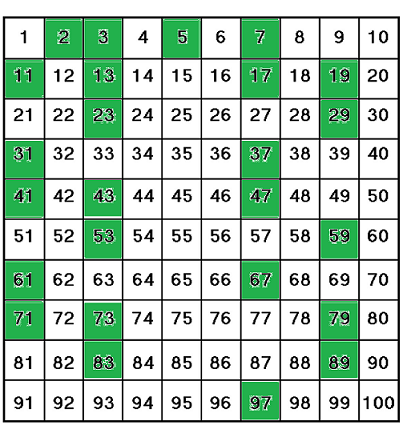# Definition of Prime NumberA prime number is a whole number that has exactly two factors: itself and $1$.

For example, $2$ only has the factors of $1$ and $2$ (it can only be divided evenly by $1$ and $2$), so it is a prime number.

The diagram shows the prime numbers between $1$ and $100$, shaded in green.

Numbers which can be divided evenly by numbers other than themselves and $1$ are called composite numbers. For example, $15 = 5 \times 3$ is a composite number.

Note that $1$ is neither prime nor composite.

### Description

The aim of this dictionary is to provide definitions to common mathematical terms. Students learn a new math skill every week at school, sometimes just before they start a new skill, if they want to look at what a specific term means, this is where this dictionary will become handy and a go-to guide for a student.

### Audience

Year 1 to Year 12 students

### Learning Objectives

Learn common math terms starting with letter P

Author: Subject Coach
You must be logged in as Student to ask a Question.